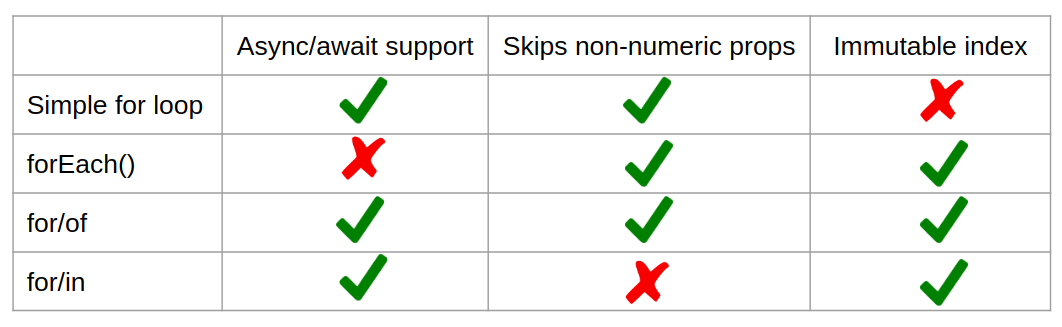# How to Iterate through an Array in JavaScript

Jul 3, 2020

There are several ways to iterate through an array in JavaScript, and there's a lot of debate about which way is the right way. Generally speaking, there are 4 common patterns:

1. Simple `for` Loop: `for (let i = 0; i < arr.length; ++i)`
2. Functional methods like `forEach()`: `arr.forEach((v, i) => { /* ... */ })`
3. The for-of loop: `for (const v of arr)`
4. The for-in loop: `for (const i in arr)`

Below are several of the most important differences between the 4 common approaches.

TLDR: Prefer to use for-of (3) where possible. Simple `for` loop (1) is OK as well. Do not use `for/in`.

## Async/Await Support

The big gotcha with functional methods like `forEach()` is that, because you pass a separate function to `forEach()`, using async/await with `forEach()` is hard. For example, the below code will print the numbers 0-9 in reverse order, because `forEach()` executes async functions in parallel and doesn't give you a way to handle errors.

``````async function print(n) {
// Wait 1 second before printing 0, 0.9 seconds before printing 1, etc.
await new Promise(resolve => setTimeout(() => resolve(), 1000 - n * 100));
// Will usually print 9, 8, 7, 6, 5, 4, 3, 2, 1, 0 but order is not strictly
// guaranteed.
console.log(n);
}

async function test() {
[0, 1, 2, 3, 4, 5, 6, 7, 8, 9].forEach(print);
}

test();``````

On the other hand, async functions work great with JavaScript's built-in loops.

``````async function print(n) {
await new Promise(resolve => setTimeout(() => resolve(), 1000 - n * 100));
console.log(n);
}

async function test() {
// Prints 0-9 in order.
for (const num of [0, 1, 2, 3, 4, 5, 6, 7, 8, 9]) {
await print(num);
}
}

test();``````

## Non-Numeric Properties

JavaScript arrays are objects. `typeof [] === 'object'`. That means arrays can have non-numeric properties. The primary reason to avoid using `for/in` is that `for/in` iterates over non-numeric properties, whereas `for`, `forEach()`, and `for/of` skip non-numeric properties.

``````const arr = ['a', 'b', 'c'];

for (let key in arr) {
console.log(arr[key]); // Prints: a, b, c, alpha
}

// However, `for/of` skips non-numeric keys
for (const val of arr) {
console.log(val); // Prints: a, b, c
}

// So does `forEach()`
arr.forEach(val => console.log(val)); // Prints: a, b, c``````

## `const` Iterator Key

One common mistake when using simple `for` loops is unintentionally incrementing `i`. I've lost count of the number of times I've accidentally incremented the wrong counter in a nested `for` loop.

``````for (let i = 0; i < arr.length; ++i) {
// So easy to accidentally type `++i` below.
for (let j = 0; j < arr.length; ++i) {

}
}``````

`forEach()`, `for/of`, and `for/in` have the nice advantage of being able to prevent messing up the loop by accidentally modifying the loop index. With `forEach()`, you can modify the `index` argument, but that has no affect on the loop. With `for/of` and `for/each`, you can mark the iterator key as a `const`.

``````for (const [i, el] of Object.entries(arr)) {
++i; // Compile time error
}``````

## Summary

Below is a chart comparing the looping constructs:You should prefer to use `for/of` unless you have a good reason not to. You may want to use `forEach()` for some neat syntactic sugar with `filter()` and `map()`, or you may actually want to loop through non-numeric properties on an array and use `for/in`. But `for/of` is the most robust approach and works well for almost all cases.

Did you find this tutorial useful? Say thanks by starring our repo on GitHub!